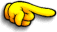Search the site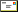Email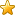Bookmark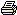Print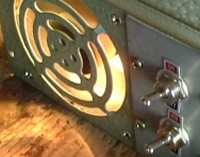Building a 300 200 watt dump load. Page 1 | 2 | 3

To put it simply, a dump load is a device that wastes power. Its used when you have an excess of power that you need to get rid of in a safe manner. We use dump loads when our battery bank is fully charged and we need to drain off the excess power coming from our source ( solar, wind, hydro ). We can also use a dump load to safely load a windmill, as a windmill should always see some sort of load and never allowed to free wheel.

We could use the extra power to do something useful, like heat water, but in most situations we just need a device that can drain power safely and reliably. Most dump loads turn electrical power into heat, and depending on the size of the dump load, need to some way to dissipate this heat.

Turning electrical power into heat is a simple task, we use something called a resistor ( symbolor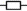). When we apply a voltage across a resistor, it draws a current from our voltage source.

The current flowing through a resistor is worked out with ohms law. If we know any two variables, we can work out the third.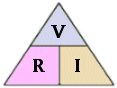Where : V = Volts R =Resistance in Ohms I = Current in Amps Volts = ohms X Amps Amps = Volts / Ohms Ohms = Volts / Amps

So, for example, if we have a 2 ohm resistor, and apply 12 volts, it will draw 6 amps. If I needed to dump 10 amps, I can work out what resistance I need for my dump load using 12 volts / 10 amps giving a 1.2 ohm resistor. Easy, go and buy a 1.2 ohm resistor.

Not so fast! That 1.2 ohm resistor will drain 10 amps from your 12 volt supply, but where does that 10 amps go? Answer - Heat! And lots of it.

Heat is power, and we measure power in Watts. To work out Watts, we use....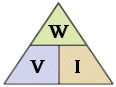Where : W = Watts V = Volts I = Amps Watts = Volts X Amps Volts = Watts / Amps Amps = Watts / Volts

Back to our 1.2 ohm resistor. At 12 volts its soaking up 10 amps, so 12 volts X 10 amps is 120 watts. The resistor needs to dissipate 120 watts of heat into its surroundings. Is 120 watts a lot? That depends on how big the area dissipating the 120 watts is. If its as big as a fry pan, you could put you hand on it and it would feel warm. If its the size of a grain of salt, it will melt steel and vaporize timber ( this is how a 300 watt laser cutter can cut 3mm steel plate, the energy is focused to a fine point. Ever used a magnifying glass to burn paper?). So as a rule, the bigger the resistor, the more heat ( watts ) it can dissipate.

Commercial resistors are designed to dissipate a certain amount of heat. The little resistors in you pocket radio will dissipate 1/4 of a watt, not much, we would need 480 little resistors to dissipate our 120 watts. Fortunately we can get higher wattage resistors.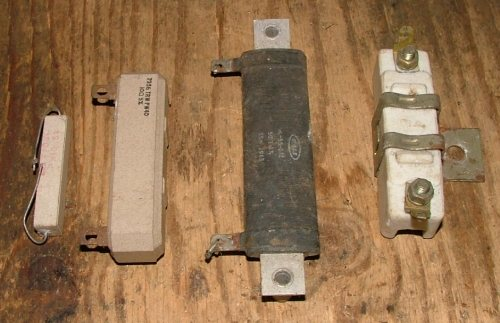From left to right we have a 10 watt, 40 watt, and two 50 watt resistors. The resistor to the far right is a ballast resistor from a car. To dissipate our 120 watts, we could use 3 of the 40 watt resistors, or better still, 3 of the 50 watt resistors, to give us some breathing space. Its a good idea to have a higher rated resistor then we need because the watts will go up if the voltage goes up. We calculated the wattage based on 12 volts, but if the source voltage went up to 13 volts, we would need to dissipate 140 watts. While we can use as many high watt resistors as needed, they can get expensive. What cheaper options are there?

 Wire Resistors... Steel wire has a higher resistance than copper or brass. We can use lengths of steel wire as a resistor. You can make your own wire resistors by winding a length of steel (fencing) wire around a former. If you know what resistance you need, use a multi meter to measure out a length of steel wire that matches the resistance. Then wrap it around a former to make it more compact and hold its shape. The resistor bottom right is wrapped around a fibreboard former. A former is used because as the wire heats up it becomes soft, and the current flowing through the wire will act like a electromagnet, which can distort the coil if its hot enough or the current is high enough. Its important to use a former that can handle the heat, it all depends on how large the resistor is and how many watts it needs to dissipate. Another way to build your own wire dump resistors is to modify heater elements designed for 240/110 VAC operation. To see how, click here.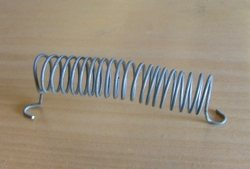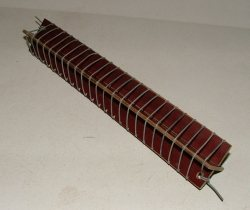Building your own high watt resistor.

The dump resistor below was made from 3 electric jug elements. The wire resistance on each element was 10 ohms, and 10 ohms across 12 volts only draws 1.2 amps. I unwound the heater wire and cut into 4 even lengths, where were then joined up in parallel before re-winding onto the ceramic insulator. This gave a resistance of 2.5 ohms, which would draw 4.8 amps, at 50 watts. There are 3 elements connected in parallel, so this contraption ended up a 150 watt dump load. It gets very hot, I should have it covered.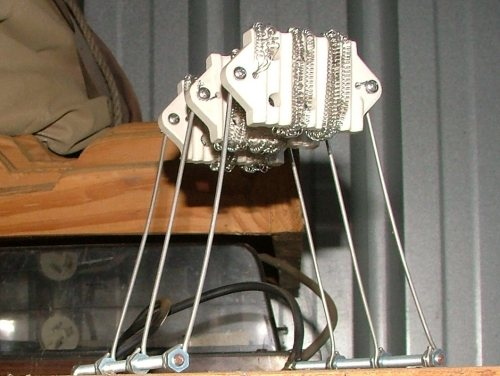Light Bulbs...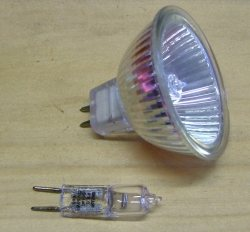Any light bulb can be used as a dump load. 12 volt bulbs are cheap, and you can wire 2 in series for 24 volts. A advantage of light bulbs is you already know what their wattage is. A 50 watt bulb will draw 50 watts, or 50w/12v = 4.1amps. There are however a couple of problems with using light bulbs that we need to consider. First up, light bulbs have a in rush current that can be several times the normal running current. When the filament is cold, it has a very low resistance compared to hot, so from a cold switch on there is a spike of current as the filament reaches its normal running temperature.

This spike only lasts a few milliseconds, but it could still be long enough to damage the switching circuit.

The other concern is a light bulb can blow if the input voltage is too high. A blown bulb is open circuit, and no longer provides any form of dump load protection to your battery bank or windmill.

Next Page - Building my 300w dump load.